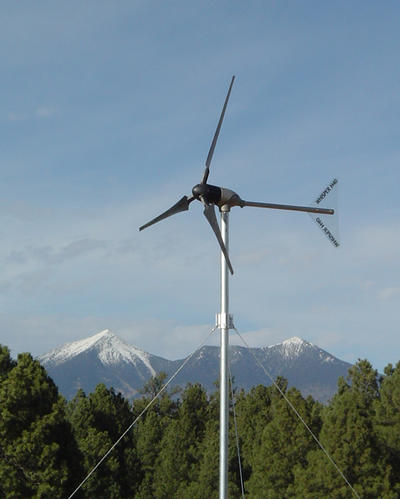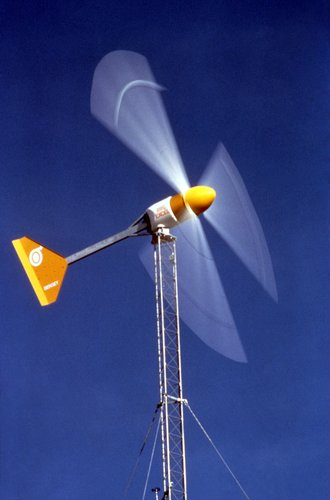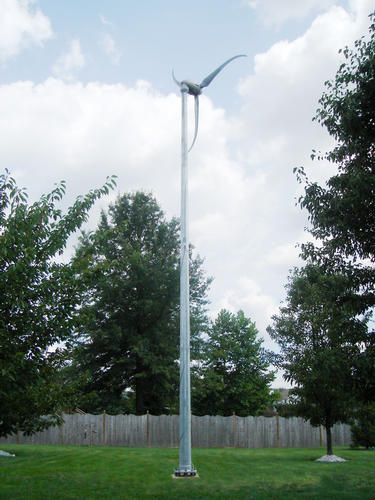﻿ glossary of small wind termshome | about us | contact us | order WindCheckglossary

 a b c d e f g h i j k l m n o p q r s t u v w x y z

Absolute humidity.  The mass of water vapor per unit volume of space, commonly measured in grams per cubic meter. The humidity of a volume of air affects the density of that volume and therefore its wind power potential.

Alternating Current (AC).   A type of electrical current in which the flow of electricity reverses direction at a periodic frequency, most commonly 60 times per second (60 hertz) in the United States. Most household electricity is AC.

Air density.   The mass of air per unit volume of space. The wind power potential of a moving volume of air is proportional to its air density.Anemometer.   An instrument designed to record wind speed. Commonly used to measure observed wind conditions at a location as a means of assessing its potential for wind power productions.

Annual Energy Output.   The energy produced over the course of a year by a specific wind turbine. Commonly used to compare the performance of one wind turbine to another.

Average wind speed.   The statistical average wind speed over a specific period of time. Used to compare the relative windiness between two locations.

Betz' Law.   The theorum advanced by German physicist Alfred Betz that no more than 59.3% of the kinetic energy of a parcel of moving air (wind) can be converted into mechanical energy via the rotors that are found in a wind turbine. This is the theoretical maximum efficiency for a wind turbine.

Braking System.   A system (electrical or mechanical) used to slow the rotation of a wind turbine.

Cut-in wind speed.   The speed that a wind turbine must reach in order to begin producing energy.

Decibel (dB).   A logarithmic unit of measurement used to compare the magnitude of one physical quantity to a reference quantity. Commonly used to express the sound levels associated with a wind turbine. Because this uses a logarithmic scale, a sound that is 3 db louder than another one is approximately twice as loud as the reference source.

Direct Current (DC).  A type of electrical current in which the electricity flows only in one direction, such as the current produced by batteries.

Drag.   That component of force generated by the flow of an object through a fluid that acts opposite to the direction of motion, such as the drag exerted on an airplane wing or wind turbine rotor by the air through which it is moving. In a wind turbine, the energy spent to overcome high drag reduces the energy available for conversion to electricity, so low drag is desirable.

Efficiency.   The ratio of an output quantity to an input quantity, such as the amount of energy leaving a wind turbine as electricty versus the amount of energy coming in to it as wind.

Electrical Grid.   The national system of transmission and distribution used to transport electricity from producers to end users. Also called "the grid".

Grid-tie inverter (GTI).A type of inverted (see below) that converts the DC electrical current generated by a wind turbine into AC electrical current that can be introduced into the grid.

Horizontal Axis Wind Turbine (HAWT).   A wind turbine in which the rotor shaft (see below) is parallel to the ground.

Inverter.   An electrical device that converts Direct Current (DC) to Alternating Current (AC).Kilowatt (kw).   A measure of power (energy per unit of time) equal to 1,000 watts of energy per second, commonly used to quantify the rate of energy generated (or consumed) per unit of time. 1 watt of power is defined as the rate of 1 joule of energy per second.

Kilowatt Hour (kwh).   A measure of energy equal to 1,000 watts of energy per second for a one hour period, commonly used to quantify the amount of energy generated (or consumed) over a specifed period of time.

Laminar flow.   The flow of air (or other fluid) which is "smooth" and without signficant variation in wind speed or direction. Laminar air flow is steady and consistent, so wind turbines are more efficient at converting laminar flow into energy than they are at converting rough (turbulent) air flow. Consequently, it is common practice to try to place wind turbines in locations that are characterized by laminar flow and distant from possible sources of turbulence.

Lift.   That component of force generated by the flow of an object through a fluid that acts perpendicular to the direction of motion, such as the lift generated by an airplane wing or wind turbine rotor when it moves through the air. In a wind turbine, the lift generated by a moving blade serves to increase the rotational speed of the rotors, thereby increasing the amount of electricity generated, so a large amount of lift is desirable.

Power Curve.   A graphical depiction that shows the power output of a wind turbine generator as a function of the wind speed.

Power Output.   The amount of power produced by a wind turbine generate for a given wind speed.

Rated Power.  The amount of power produced by a wind turbine generate at a selected wind speed, known as the Rated Wind Speed. If the Rated Wind Speed is the same between two wind turbines, consumers can look at the Rated Power to see which turbine will generate more energy for that selected wind speed. Important caution: manufacturers often choose a wind speed that is relatively high to calculate their rated power. However, since the average wind in many locations is much less than that rated wind speed, the turbine ends up generating far less than the rated power.

Rated Wind Speed.   The selected wind speed at which a wind turbine's rated power is calculated.

Rayleigh distribution.   A statistical distribution of physical values over a spectrum of possible values, commonly used to describe the distribution of wind speeds in nature. The Rayleigh distriubtion is less accurate but simpler to calculate than the related Weibull distribution.

Relative Humidity.   The ratio of the partial pressure of water vapor in a parcel to the vapor pressure of water if that parcel were saturated, expressed as a percent (0-100%). Commonly used as a measure of the density of a volume of air (warm moist air is less dense than cold dry air) and therefore related to the energy that can be generated by a wind turbine.

Rotor.   The rotating blades on a wind turbine.

Rotor Shaft.   The rotating shaft to which the rotating blades of a wind turbine are attached. The rotation of the Rotor Shaft as part of a generator assembly is what allows the rotational energy of the blades to be translated into mechanical and then electrical energy.

Rotor Speed.   The rotational speed of a wind turbine rotor, commonly expressed in revolutions per minute.Swept Area.   The area of the atmosphere that is swept by the rotors of a wind turbine, commonly expressed in square meters or square feet. The power output of a wind turbine is directly related to the area swept by its blades, so longer blades lead to greater power generated.

Turbulent Flow.   The "rough" and uneven flow of air (or other fluids), characterized by irregular variations in wind speed and wind direction. Turbulent air flow is inconsistent and chaotic, so wind turbines are less efficient at converting turbulent air flow into energy than they are at converting smooth (laminar) air flow. Consequently, it is common practice to try to place wind turbines as far away from sources of turbulence as possible.

Vertical Axis Wind Turbine (VAWT).   A wind turbine design in which the rotor shaft is perpendicular to the ground.

Watt.   A measure of power (energy per unit of time), defined as the rate of 1 jouble of energy per second. Commonly used to quantify the amount of energy generated (or consumed) per unit of time.

Weibull Distribution.   A statistical distribution of physical values over a spectrum of possible values, commonly used to describe the distribution of wind speeds in nature. The Weibull distribution is more accurate but harder to calculate than the related Rayleigh distribution.

Wind Direction.   In meteorological convention, the compass direction that winds are blowing from. Thus a NorthEast wind is blowing from the Northeast.

Wind Turbine.   A mechanical device that converts the kinetic energy of the wind into mechanical energy used to move some sort of machinery (which could be an electrical generator).